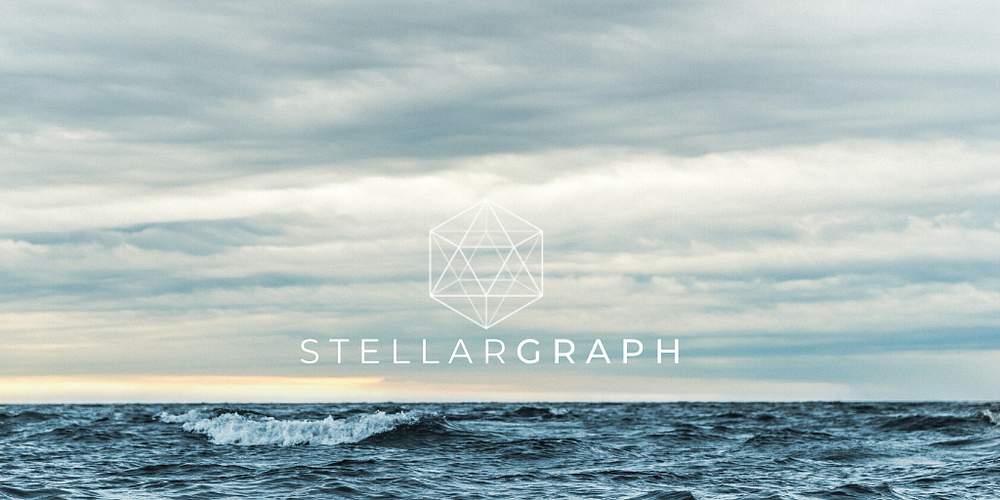# Embedding the structural properties of nodes: Riding the GraphWave.

Original article was published on Deep Learning on Medium## How does GraphWave work?

Let’s begin by demonstrating how GraphWave addresses a deficiency in other algorithms; that being the inability to capture the structural similarities of nodes.

The plot below (Figure 2) shows how the Node2Vec algorithm encodes the graph at Figure 1, and its limitations. The nodes at the left and right cluster are far apart from each other which renders them far apart in the Node2Vec embedding space, despite the structure around these nodes being visually very similar.

The GraphWave algorithm is a way to capture the structural similarities of the nodes.

To exemplify this, consider a sound signal travelling from the bottom left node. This signal travels around the graph and will be louder at closer nodes, and quieter at more distant nodes. Figure 3 shows what this might look like with “louder” nodes coloured darker, and Figure 4 is a histogram of these volumes:

If the sound signal originates from the bottom right node instead, the volume at each node will be different. However, because this node has the same number of neighbours, and each of those neighbours has the same number of neighbours (and so on), when we look at the histogram of volumes, it’s identical:

The basic idea of GraphWave is to diffuse signals throughout the graph for each node, and encode the diffused signal histograms as vectors (Figure 7). In the case of our example graph, the embeddings of structurally similar nodes almost completely overlap.

## The mathematics

GraphWave leverages spectral graph theory to calculate the diffusion of signals across the graph. Spectral graph theory examines the eigenvalues, eigenvalues of the graph adjacency and Laplacian matrix to derive information about the graph. In our case we’re interested in the graph Laplacian matrix, defined as: